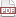### Bayesians and ABMs

21 July 13. [link]PDF version

Part of a series of posts that started here, riffing on this paper.

$\def\Re{{\mathbb R}} \def\datas{{\mathbb D}} \def\params{{\mathbb P}} \def\models{{\mathbb M}} \def\mod#1{M_{#1}} \def\muv{\boldsymbol{\mu}} \def\Sigmav{\boldsymbol{\Sigmav}}$

Let us say that our model is a deterministic formula $f:\params\to\datas$ (or $g:\datas\to\params$). The likelihood function $$L(d, p) =\left\{\begin{matrix} 1, & d = f(p)\\ 0, & \textrm{all other cases} \end{matrix}\right.$$ is … unsatisfying. A few entries ago, I presented a variant of linear regression with the convenient feature that it rejects the null hypothesis of $\beta=0$ with probability one. It was dissatisfying (partly) because it was artificially overconfident; using a deterministic function as a likelihood, claiming that $L(f(p), p) = 1$ but $L(f(p)+\epsilon, p)=0$, is even more unsatisfying for the same reason.

We accommodate uncertainty in a few ways.

One is to add a loss of some sort. Given observed data $d_{obs}$, define $\Delta \equiv |f(p) - d_{obs}|$. We could define the likelihood $L(\Delta)$ as:

• $\frac{1}{1+\Delta}$
• $\frac{1}{1+\Delta^2}$
• for fixed $\alpha > 0$, $\left\{\begin{matrix} 1-\alpha\Delta, & \Delta \leq 1/\alpha \\ 0, & \Delta > 1/\alpha \end{matrix}\right.$
• $\exp(-\Delta)$
• $\exp(-\Delta^2)$

These all share the intuitive characteristic that they are largest at $\Delta=0$ and get smaller as $\Delta\to\infty$. You could easily come up with many other functions that share this basic requirement.

For the first loss function, $1/(1+\Delta)$, the integral from $\Delta=0$ to $\Delta=k$ is $\log{1+k}$, which goes to infinity as $k\to\infty$; that makes it inadmissible as a likelihood function.

For the others, it is increasingly a judgment call as to which is preferable. The consensus choice is of course $\exp(-\Delta^2)$, because there are central limit theorems that posit that this is the outcome from a sequence of iid draws being averaged together.

In case I'm not clear here, ordinary least squares is the prime example of this form: let the data be decomposable into independent and dependent components $[Y X]$; then $f([Y X]) = (X'X)^{-1}X'Y \equiv \beta$, and we assume the loss $\Delta \equiv Y-\beta X$ is Normally distributed.

Textbooks will typically put some verbiage in here about how, if you have the `correct' model, then the errors should be Normally distributed white noise. They will then search a subspace of measure zero within the space of models for the correct model. This setup is perfectly reasonable and plausible, but do note that the design right from the start is that we have some theory about $f(\cdot)$, and $\Delta$ is outside of that theory, so it really provides no information about its distribution. The deterministic function that is the core of the model and the distribution of $\Delta$ are separated by construction.

###### The Agent-based alternative

A few posts ago, I discussed the idea of using an RNG as the basis of a model, deriving the likelihood from the RNG. Last time, I presented the example of a simple agent-based model to show how that RNG can be a simulation that explicitly describes decisions, interactions, and aleatory behavior within the steps of a narrative. In the simple demand-side example from last time, the price $p$ was a parameter of the model, and agents randomly receive coefficients $\alpha$ and $b$ and then pick quantities $q_1$ and $q_2$ to maximize utility $U=q_1^\alpha + q_2$ subject to budget constraint $b=p q_1 + q_2$. A Normally-distributed $\alpha$ or $b$ would translate into distributions on $q_1$ and $q_2$.

Once again, we've found a way to produce a distribution that is not deterministic or that otherwise makes it trivial to reject a null hypothesis. We've acknowledged that the world is uncertain.

But randomness has been pushed forward to the beginning of the model: we are uncertain about the coefficients. In this case, given the coefficients, there is a deterministic calculation to get to $[q_1, q_2]$, but a more complex model could easily add aleatory steps subsequent to that point. Contrast this to the deterministic component-plus-error likelihood which assumes separability from the start.

There are two points here: the first is that the ABM way of accommodating uncertainty is not necessarily more or less plausible than the traditional—let's call it Frequentist—way of doing things. We just put the uncertainty earlier in the model and reserve the option to have as many random steps as our narrative requires.

The second point is that when we talk about parameters of the model having uncertain terms, we are fast treading on Bayesian territory. The Bayesian way is also to push randomness to the beginning of the model. The typical Bayesian textbook presents a likelihood function that is a typical textbook distribution, and then allows its parameters to vary according to a prior distribution; while our demand-side example presents a likelihood function defined by a narrative description, such as a simple utility maximization model, and then allows its parameters to vary according to a set of distributions.

Next time, I'll present a worked example.